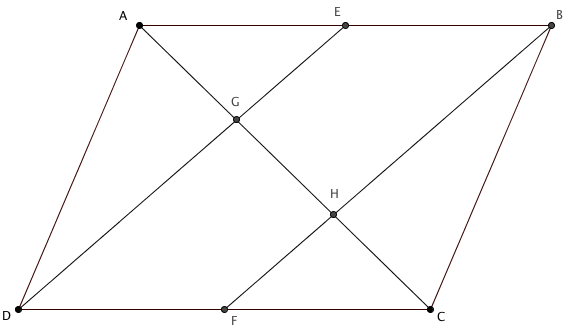# Parallelo-split

##### Age 14 to 16Challenge Level

$ABCD$ is a parallelogram.

A diagonal, $AC$, has been drawn, as well as two lines $ED$ and $FB$, from the midpoints of sides $AB$ and $CD$, respectively, to opposite vertices.Draw a parallelogram and construct the lines shown above. You may wish to use GeoGebra.

What do you notice about the lengths $AG$, $GH$ and $HC$?
Is it true for all parallelograms?
Can you prove it?

Extension

Can you split the diagonals of other quadrilaterals in a similar way?